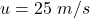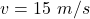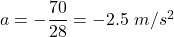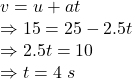## A force of 70 N is applied to a 28 kg rock causing it to slow down from 25 m/s to 15 m/s, a change in velocity of 10 m/s. How long was that

Question

A force of 70 N is applied to a 28 kg rock causing it to slow down from 25 m/s to 15 m/s, a change in velocity of 10 m/s. How long was that force applied?

in progress 0
5 months 2021-08-19T19:40:03+00:00 1 Answers 0 views 0

## Answers ( )

1. Answer: 4 s

Explanation:

Given

The applied force is 70 N

mass of the rock is 28 kg

initial velocityfinal velocityDeceleration provided by force isusing the equation of motion